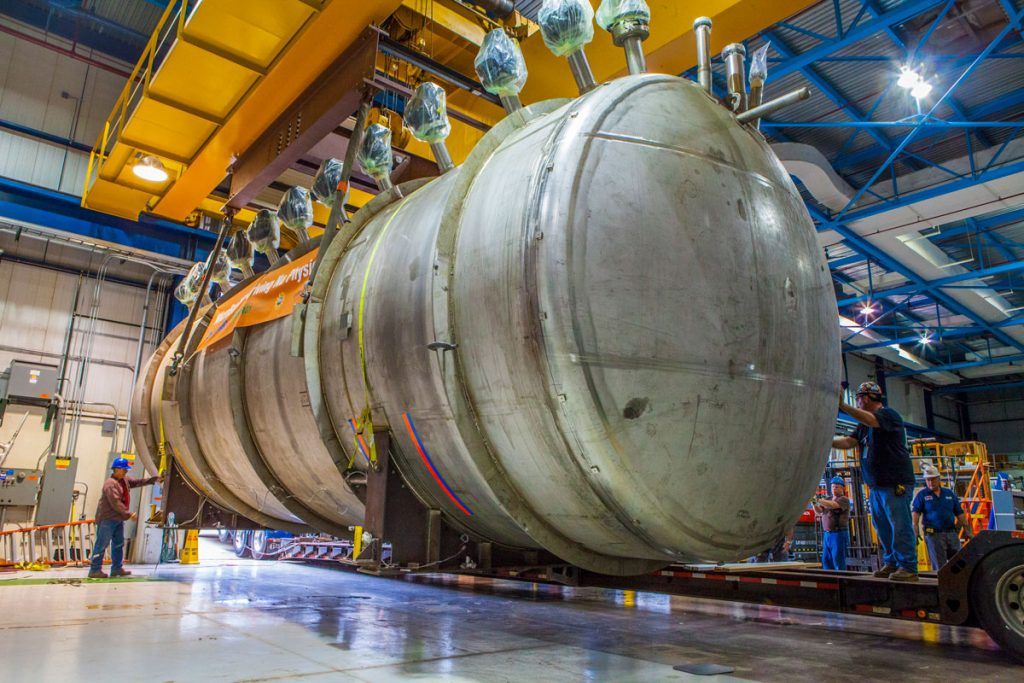# Sterile neutrinos

Sterile neutrinos are a special kind of neutrino that has been proposed to explain some unexpected experimental results, but they have not been definitively discovered. Scientists are looking hard for them in many different experiments.

While the standard electron, muon, and tau neutrinos (and antineutrinos) interact with matter through two forces (the weak force and gravity), scientists think sterile neutrinos might interact only through gravity. This would make them even harder to spot than the tricky “regular” neutrinos. Gravity is the weakest of all the forces, and neutrinos are very light—so they don’t give gravity much to work with. Finding slight signals amidst the chaos of the universe will be tough, but not impossible.

While they know of the three flavors of neutrinos, scientists aren’t sure how many kinds of sterile neutrinos there might be. Is there just one to add in, or perhaps a parallel three? Or maybe there are even more!

Particles (including the wacky neutrino) have properties called spin and helicity. The particles don’t literally spin like a top, but this is still a good way of thinking about it. Helicity refers to how the spin relates to the movement of the particle, and it’s analogous to the idea of someone being left handed or right handed.

Hold out your hands and make two fists. The way your fingers curl represents a particle’s spin, and your thumb points in the direction of travel. These are right-handed and left-handed particles, and they’re important because one of nature’s four forces—the weak force—does not treat them equally. The weak force strongly prefers to interact with left-handed particles.

So far, scientists have found only left-handed neutrinos. But if there are right-handed neutrinos, they could be the predicted sterile neutrinos. Because the weak force would ignore them, sterile (right-handed) neutrinos would interact only through gravity, making them borderline invisible.

One way to discover these secretive particles involves oscillation. Some experiments have seen an excess neutrino oscillation where theory predicted they shouldn’t be. And some experiments have seen neutrinos appearing or disappearing over much shorter distances than the experiments on neutrinos from more distant locations, such as the atmosphere or sun. If neutrinos oscillate into this fourth kind of neutrino, that could explain the rapid changes and the anomalies seen in experiments. Much more data is needed before anything can be decided definitively.

Neutrinos interact through two of the four Standard Model forces: the weak force and gravity. It is this lack of interactions (and their tiny mass) that gives them their ghostly nature. For example, 50 percent of the neutrinos coming from the sun will pass through a light-year of lead without interacting.

But imagine a neutrino, already nearly massless, that does not interact through the weak force. How would scientists even know they were there? This is exactly the challenge with searching for “sterile” neutrinos.

At the Large Electron-Positron (LEP) collider at CERN, scientists measured the particles that emerged from collisions between electrons and positrons. One particle, the Z boson, is the carrier of the weak force, and how quickly it decays depends strongly on the number of particles that it couples to. By measuring the decays of the Z boson, scientists were able to measure to a very high precision that only three neutrinos couple to the weak force: the electron, muon and tau neutrinos. But there could be any number of extra “sterile” neutrinos that LEP would be unable to see—though scientists would still need to figure out why.

The hints of sterile neutrinos come from a couple of experiments. The Liquid Scintillator Neutrino Detector (LSND) experiment at Los Alamos National Laboratory studied a decay-at-rest beam made of mainly muon neutrinos and found more electron neutrinos than they predicted. This was a similar signature of oscillation that had been seen for the known neutrino flavors, but at a distance and energy combination researchers weren’t expecting. A similar signal at a new location is a hint that an unknown kind of neutrino was hiding behind the scenes.

There is a lot of ongoing work to confirm if this interpretation of the LSND results is correct. So far the results have been inconclusive from these experiments. The MiniBooNE experiment at Fermilab saw hints that could also be interpreted as extra electron neutrinos appearing due to the existence of the sterile neutrinos, but the MINOS experiment, Daya Bay Reactor Neutrino Experiment, and other projects looking for neutrinos disappearing due to sterile neutrinos did not see that signal. The Short Baseline Neutrino program at Fermilab will use three liquid-argon detectors that are currently running or under construction. This suite of projects aims to definitively answer this question by making very high-precision measurements of a muon neutrino beam produced at Fermilab.The MicroBooNE detector is one of the three short-baseline neutrino detectors at Fermilab. It is hunting sterile neutrinos and testing the liquid-argon technology that will be used for the enormous Deep Underground Neutrino Experiment. Credit: MicroBooNE/Fermilab

While the short-baseline experiments look for light sterile neutrinos carrying a relatively small amount of energy—at the electronvolt scale—there could be different sterile neutrinos at different energies. Sterile neutrinos around 1,000 electronvolts could be related to dark matter or other cosmological issues. And neutrinos around 1013 gigaelectronvolts could be the heavy seesaw neutrinos that help explain the small neutrino masses we see in the known light neutrinos. At just above this scale, 1015 gigaelectronvolts, physicists also start talking about grand unified theories and how different forces relate to one another.

So sterile neutrinos could tie into many different elements of physics. All neutrinos that scientists have seen so far are left-handed, and all antineutrinos are right-handed, but if there were right-handed neutrinos, they could act just like sterile neutrinos. Other particles have both left- and right-handed versions, and right-handed neutrinos are a popular way of adding neutrino masses into the Standard Model.